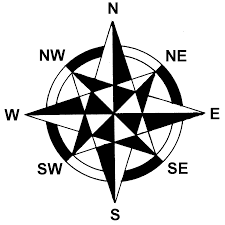• Sora03
The hiker walks 25km southeast from her base camp. When she arrives at the forest ranger's tower on her second day, she discovers that it is at a 60-degree angle from north. The hiker's displacement is 10.8 kilometers due to this angle and her speed is 75 kilometers per hour.f

## Homework Statement

I am having difficulties in analyzing problems. Here are the problems:
A1. A hiker begins trip by walking 25km southeast from her base camp. on the second day, she walks 40km in a direction of 60 degrees N of E, at which point she discovers a forest ranger's tower. Determine the components of the hiker's displacement.
A2. Consider yourself in a car (A) traveling along a straight, level highway with a speed of V(a) = 75km/h. Another car (B) travels at a speed V(b)=90 km/h. Find the differences in the velocities V(ba) = V(b) - V(a) when (a) the car travels in the same direction in front of you and (b) the other car is approaching you traveling the opposite direction,

## Homework Equations

Vector Components
sine, cosine, tangent
Pythagorean theorem

## The Attempt at a Solution

A1. Given:
A = 25km SE
B = 40km 60 deg NE
∑Fx = -25km + 40km cos60 = -5km
∑Fy = -25km - 40km sin60 = 9.64 km
R=(5^2)+(9.64^2)=10.8 arc tan (y/x) = 62.58deg

other format:
DISTANCE ANGLE x-comp y-comp
25 km - -25km ? -25km? (should this be here or not)
40 km 60 20 km 34.64 km
is my answer right,? do I have to add the 25km as a component for x and y,it does not have any angle but only a direction (SE)

and is the 'Theta' always in the 'x-axis' not in the 'y-axis' also having difficulties in identifying theta.

A2. I didn't really understand this problem, how can I use vectors to solve it.?